#### Linear AlgebraTowards Data Science

Algebra is firstly taken from a book, written by Khwarizmi(780-850 CE), which is about calculation and equations. It is a branch of mathematics in which letters are used instead of numbers. Each…

Read more at Towards Data Science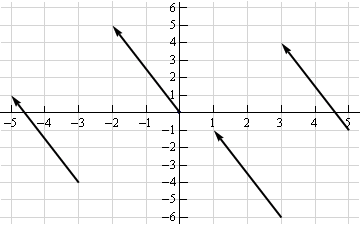#### Linear AlgebraMachine Learning Glossary

Linear Algebra Vectors Notation Vectors in geometry Scalar operations Elementwise operations Dot product Hadamard product Vector fields Matrices Dimensions Scalar operations Elementwise operations Had...

Read more at Machine Learning Glossary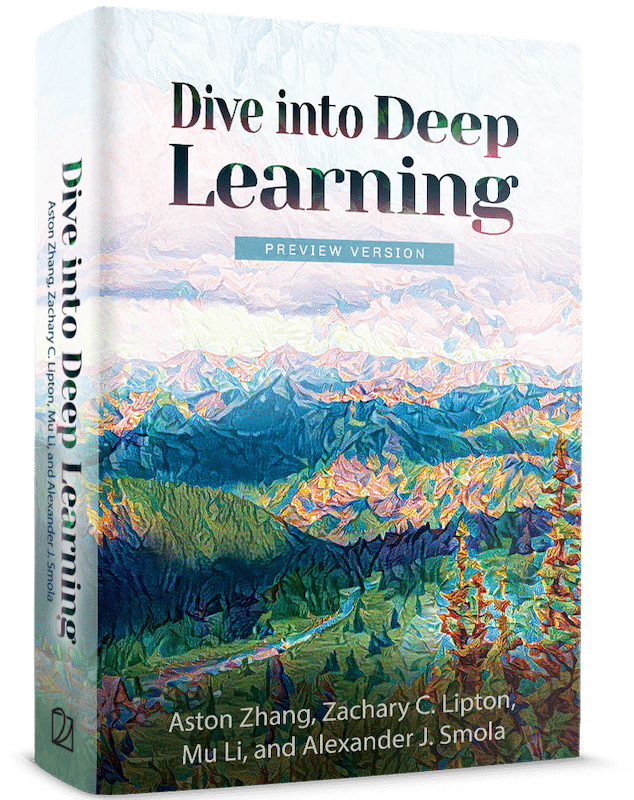#### Linear AlgebraDive intro Deep Learning Book

By now, we can load datasets into tensors and manipulate these tensors with basic mathematical operations. To start building sophisticated models, we will also need a few tools from linear algebra. Th...

Read more at Dive intro Deep Learning Book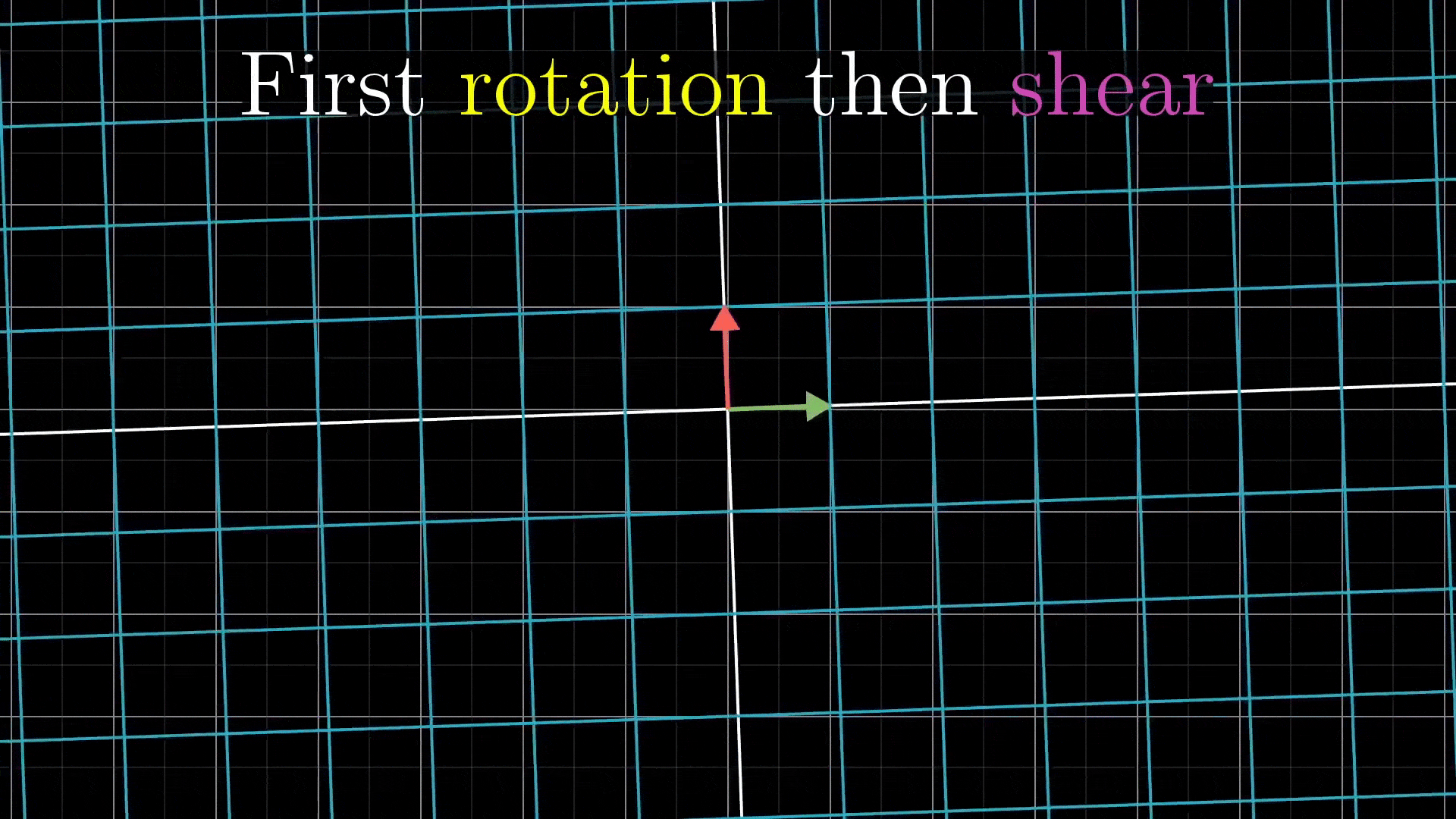#### Linear Algebra Explained — Part 2Analytics Vidhya

In the first part of this series vectors, basis vectors, span and linear transformations are explained. For this part, we will take a look at matrix multiplication, determinant, inverse matrices…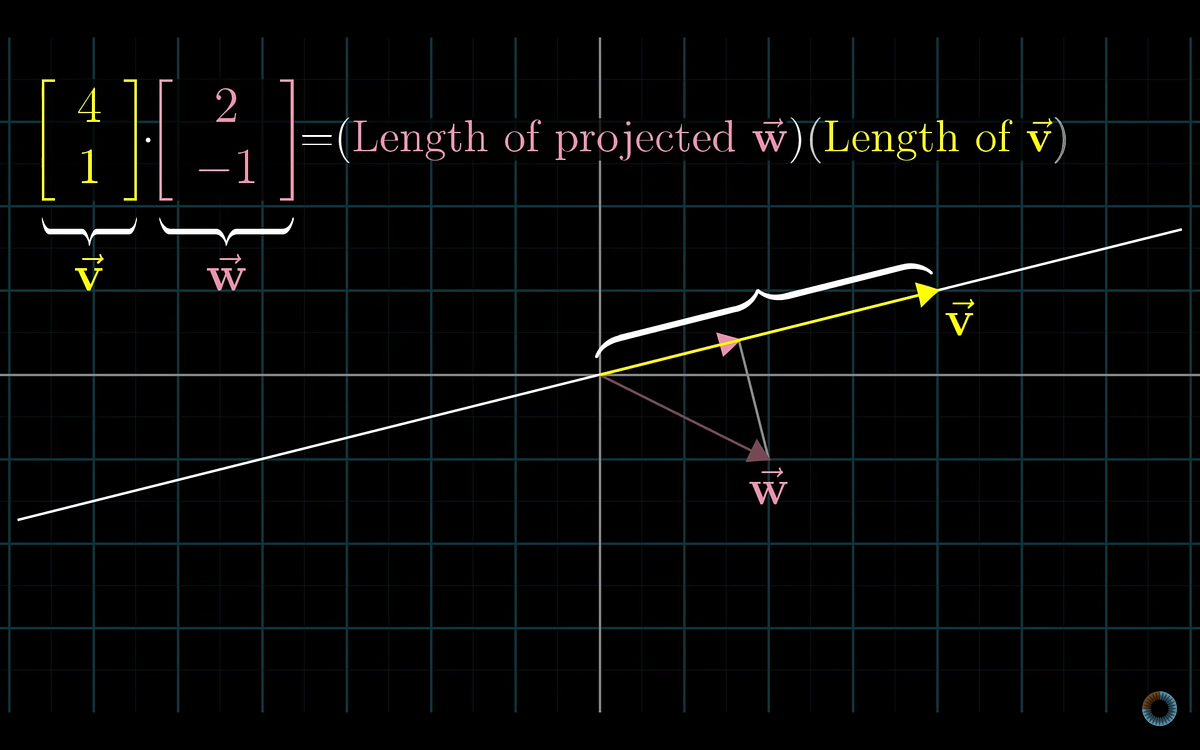#### Linear Algebra Explained — Part 3Analytics Vidhya

In this post, we will try to understand the dot product. You can read the previous posts of this series here: part 1, part 2. Let’s start with the standard definition of dot product. We take two…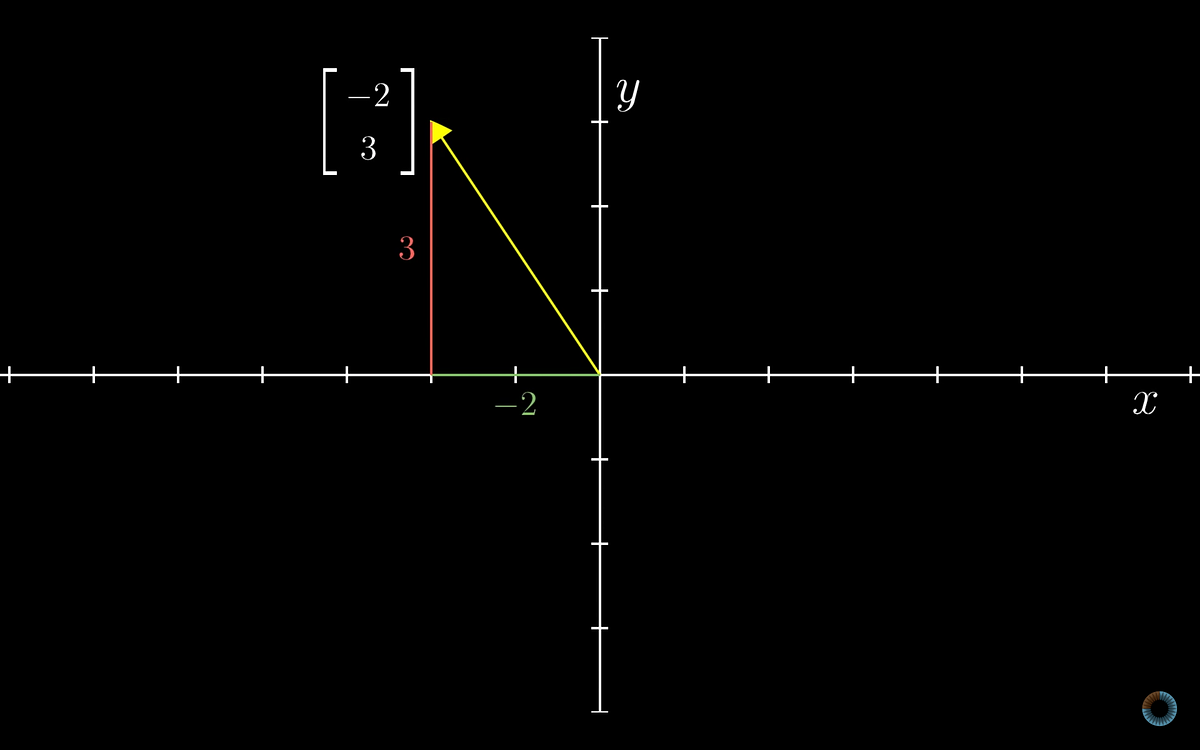#### Linear Algebra Explained — Part 1Analytics Vidhya

Linear algebra is required for most fields, especially for machine learning and data science. I watched a video series called Essence of Linear Algebra and I found them very helpful to understand the…...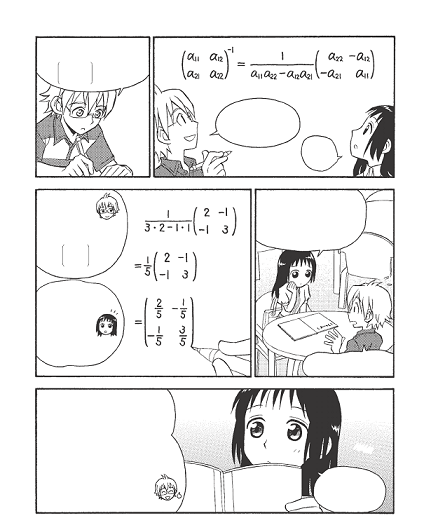#### Linear Algebra is fun, trust me! (Part 1)Analytics Vidhya

Linear Algebra is one area of Mathematics that is extensively used throughout machine learning especially in the field of deep learning. A good understanding of this area is required for…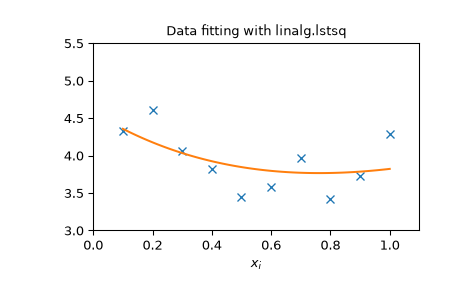#### Linear Algebra (SciPy User Guide

Linear Algebra ( scipy.linalg ) When SciPy is built using the optimized ATLAS LAPACK and BLAS libraries, it has very fast linear algebra capabilities. If you dig deep enough, all of the raw LAPACK and...

Read more at SciPy User Guide#### A Gentle Introduction to Linear AlgebraMachine Learning Mastery

Last Updated on August 9, 2019 What is Linear Algebra? Linear algebra is a field of mathematics that is universally agreed to be a prerequisite to a deeper understanding of machine learning. Although ...

Read more at Machine Learning Mastery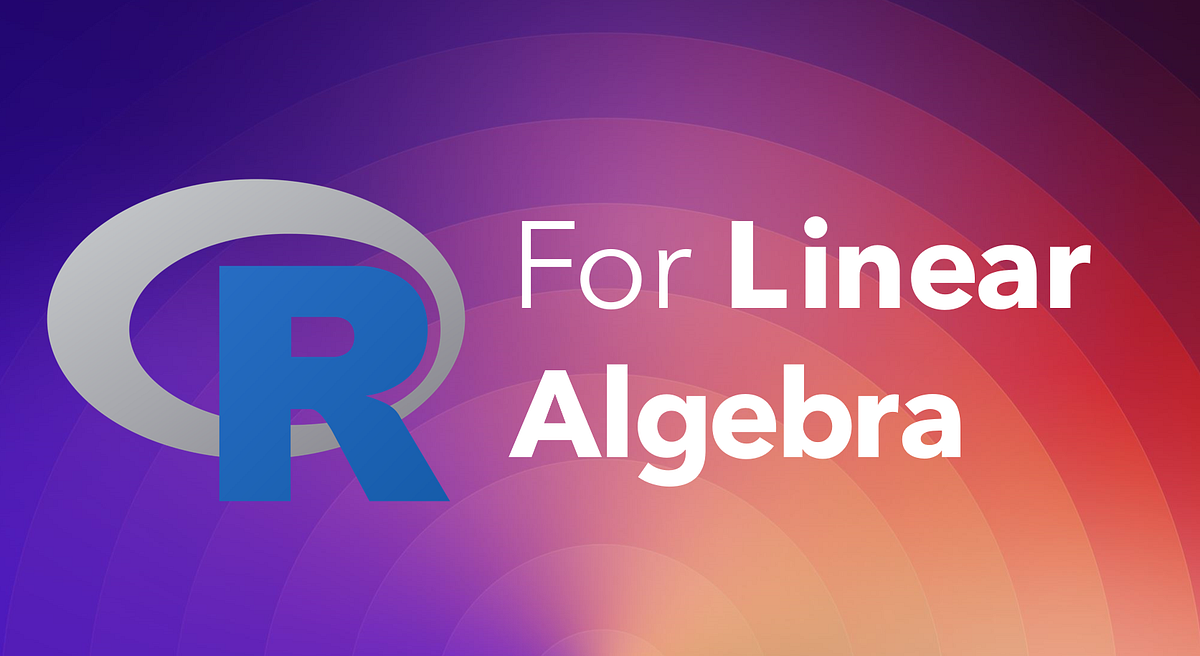#### Intro to R: Linear AlgebraTowards Data Science

R is a very powerful language developed especially for data analysis, data visualization, and machine learning, which makes it a must-learn for any aspiring data scientist. R is especially handy with…...

Read more at Towards Data Science#### Introduction to Applied Linear Algebra: VectorsTowards Data Science

Goal: This article gives an introduction to vectors, vector operations and their applications in the field of data science Why you should learn it: It is the basis for almost all machine learning…

Read more at Towards Data Science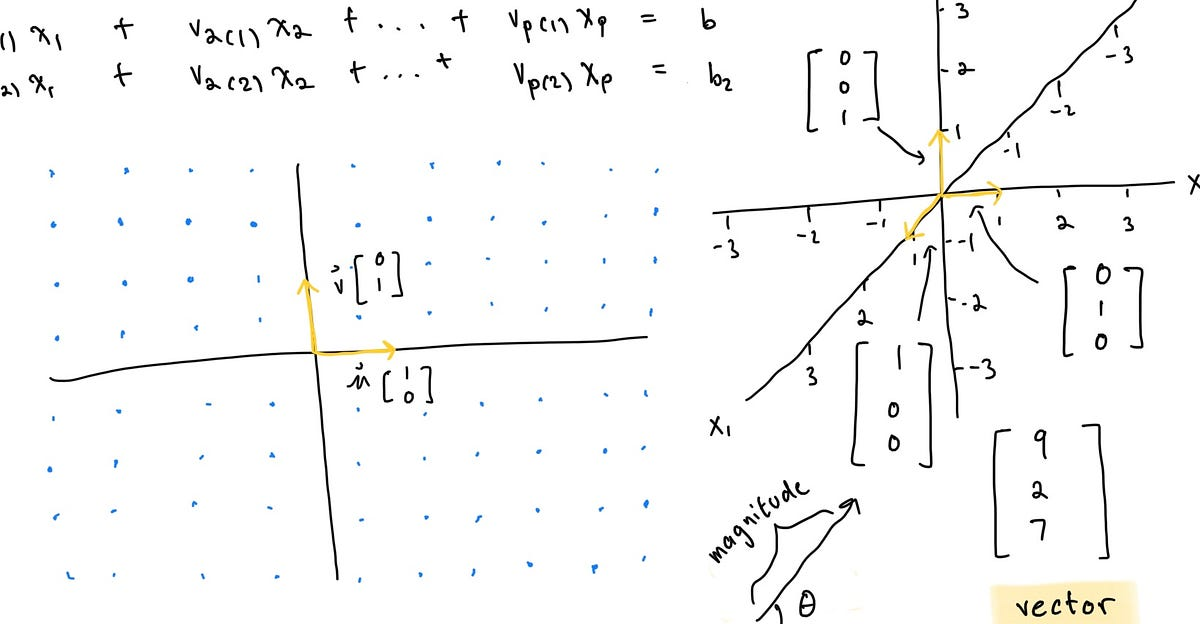#### Linear Algebra 3: Vector EquationsTowards Data Science

Vector Equations and Spans Preface Welcome back to the third essay of my ongoing series on the basics of Linear Algebra, the foundational math behind machine learning. In my previous article, I walke...

Read more at Towards Data Science#### A Primer on Linear AlgebraTowards Data Science

A gentle refresher on essential concepts and operations for data science Continue reading on Towards Data Science

Read more at Towards Data Science#### A Primer on Linear Algebra: Part 2Towards Data Science

A gentle refresher on essential concepts and operations for data science Continue reading on Towards Data Science

Read more at Towards Data Science#### Linear Algebra: General Vector SpaceAnalytics Vidhya

This article will introduce the general vector space concept and its related concepts including subspaces, basis, rank, and nullity. These concepts are important in data science by providing a mathema...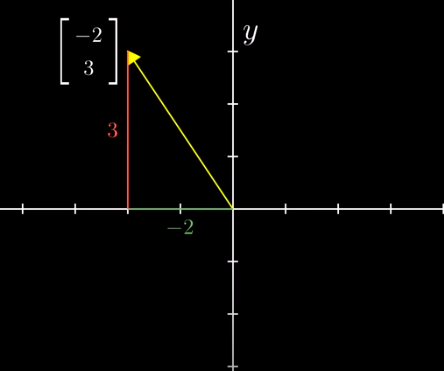#### 2 Major Concepts On Linear Algebra -SHOULD KNOW!Analytics Vidhya

Linear Algebra comes as a prerequisite for Data Science, so in this article we will study and understand few really important topics in the easiest way possible. In mathematics, a matrix is a…

#### Notes on Linear Algebra Part 1R-bloggers

If you are already familiar with much of linear algebra, as well as the relevant functions in R, read no further and do something else! If you are like me, you’ve had no formal training in linear alge...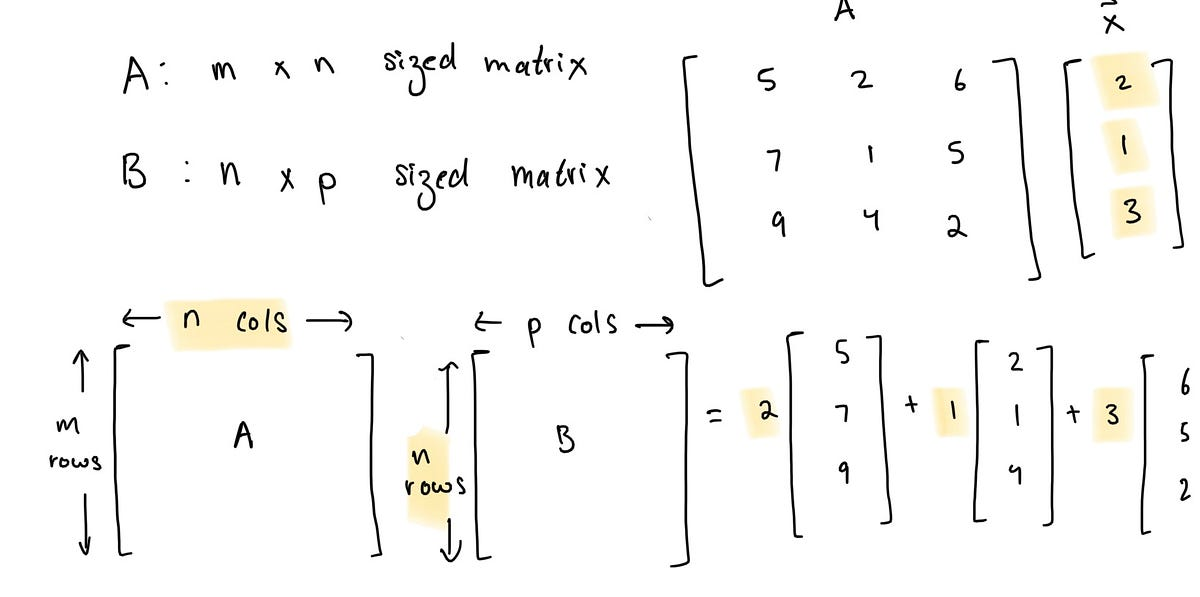#### Linear Algebra 4: Matrix EquationsTowards Data Science

Solving matrix equations Ax= b Preface Welcome back to the fourth edition of my ongoing series on the basics of Linear Algebra, the foundational math behind machine learning. In my previous article, ...

Read more at Towards Data Science

#### Notes on Linear Algebra Part 2R-bloggers

TL;DR Linear algebra is complex. We need a way to penetrate the thicket. Here’s one. Linear systems of equations are at the heart, not surprisingly, of linear algebra. A key application is linear regr...#### <h1 class="post-full-title">Linear Algebra Basics 3: Linear Transformations and Matrix Multiplication</h1>Machine Learning From Scratch

What is Linear Transformations? What is Vector Space? How to do Vector Multiplication (Matrix Multiplication)? Conceptualizing a Linear Transformation is also key to understanding a transformation, so...

Read more at Machine Learning From Scratch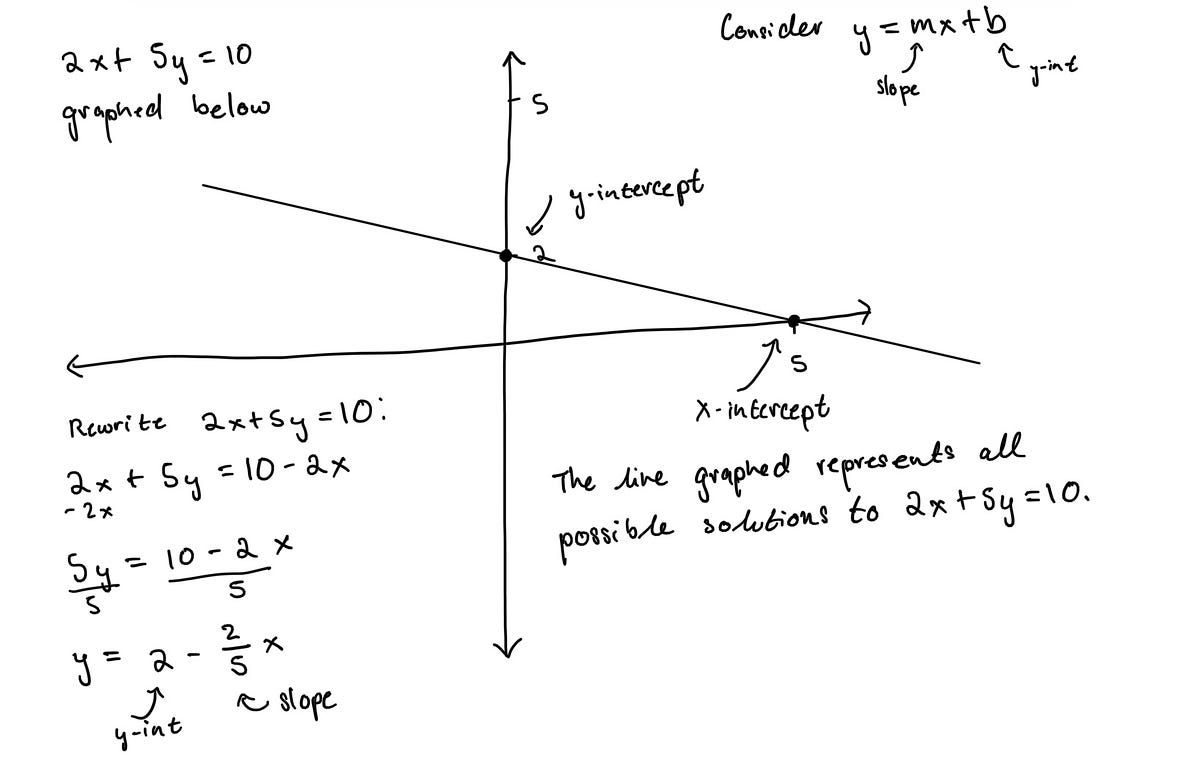#### Linear Algebra 1: Linear Equations and SystemsTowards Data Science

Image from Städel Museum Systems of linear equations Preface This is the first addition to what will become an ongoing series on the basics of Linear Algebra, the foundational math behind machine lear...

Read more at Towards Data Science#### Beginner’s Introduction to MatricesTowards Data Science

Matrices are the building blocks of data science. They appear in various avatars across languages. From numpy arrays in Python, to dataframes in R, to matrices in MATLAB. The matrix in its most basic…...

Read more at Towards Data Science#### Linear Algebra: Matrix Operations and their Properties, with PythonTowards Data Science

Part 2: Explaining the fundamental of linear algebra: matrix operations, and their properties Continue reading on Towards Data Science

Read more at Towards Data Science#### <h1 class="post-full-title">Linear Algebra Basics 1: Vectors, Vector Addition and Scalars</h1>Machine Learning From Scratch

What is a vector? How to think about vectors? What is vector addition? What is a scalar? Come find the answers here.

Read more at Machine Learning From Scratch